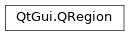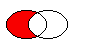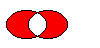# QRegion¶

The `QRegion` class specifies a clip region for a painter. More## Detailed Description¶

`QRegion` is used with `setClipRegion()` to limit the paint area to what needs to be painted. There is also a `repaint()` function that takes a `QRegion` parameter. `QRegion` is the best tool for minimizing the amount of screen area to be updated by a repaint.

This class is not suitable for constructing shapes for rendering, especially as outlines. Use `QPainterPath` to create paths and shapes for use with `QPainter` .

`QRegion` is an implicitly shared class.

### Creating and Using Regions¶

A region can be created from a rectangle, an ellipse, a polygon or a bitmap. Complex regions may be created by combining simple regions using `united()` , `intersected()` , `subtracted()` , or `xored()` (exclusive or). You can move a region using `translate()` .

You can test whether a region `isEmpty()` or if it `contains()` a `QPoint` or `QRect` . The bounding rectangle can be found with `boundingRect()` .

Iteration over the region (with `begin()` , `end()` , or C++11 ranged-for loops) gives a decomposition of the region into rectangles.

Example of using complex regions:

```class MyWidget (QWidget):
# ...
def paintEvent(self):
r1 = QRegion(QRect(100, 100, 200, 80), QRegion.Ellipse) # r1: elliptic region
)
r2 = QRect(100, 120, 90, 30)   # r2: rectangular region
r3 = r1.intersected(r2)        # r3: intersection

painter = QPainter(self)
painter.setClipRegion(r3)
...                            # paint clipped graphics
```
class PySide2.QtGui.QRegion

PySide2.QtGui.QRegion(bitmap)

PySide2.QtGui.QRegion(pa[, fillRule=Qt.OddEvenFill])

PySide2.QtGui.QRegion(r[, t=Rectangle])

PySide2.QtGui.QRegion(region)

PySide2.QtGui.QRegion(x, y, w, h[, t=Rectangle])

param w:

int

param x:

int

param y:

int

param h:

int

param fillRule:

`FillRule`

param bitmap:

`PySide2.QtGui.QBitmap`

param region:

`PySide2.QtGui.QRegion`

param r:

`PySide2.QtCore.QRect`

param t:

`RegionType`

param pa:

`PySide2.QtGui.QPolygon`

Constructs an empty region.

Constructs a rectangular or elliptic region.

If `t` is `Rectangle` , the region is the filled rectangle (`x` , `y` , `w` , `h` ). If `t` is `Ellipse` , the region is the filled ellipse with center at (`x` + `w` / 2, `y` + `h` / 2) and size (`w` ,``h`` ).

PySide2.QtGui.QRegion.RegionType

Specifies the shape of the region to be created.

Constant

Description

QRegion.Rectangle

the region covers the entire rectangle.

QRegion.Ellipse

the region is an ellipse inside the rectangle.

PySide2.QtGui.QRegion.begin()
Return type:

`PySide2.QtCore.QRect`

Returns a `const_iterator` pointing to the beginning of the range of non-overlapping rectangles that make up the region.

The union of all the rectangles is equal to the original region.

`rbegin()` `cbegin()` `end()`

PySide2.QtGui.QRegion.boundingRect()
Return type:

`PySide2.QtCore.QRect`

Returns the bounding rectangle of this region. An empty region gives a rectangle that is `isNull()` .

PySide2.QtGui.QRegion.cbegin()
Return type:

`PySide2.QtCore.QRect`

Same as `begin()` .

PySide2.QtGui.QRegion.cend()
Return type:

`PySide2.QtCore.QRect`

Same as `end()` .

PySide2.QtGui.QRegion.contains(p)
Parameters:
Return type:

bool

PySide2.QtGui.QRegion.contains(r)
Parameters:
Return type:

bool

PySide2.QtGui.QRegion.end()
Return type:

`PySide2.QtCore.QRect`

Returns a `const_iterator` pointing to one past the end of non-overlapping rectangles that make up the region.

The union of all the rectangles is equal to the original region.

`rend()` `cend()` `begin()`

PySide2.QtGui.QRegion.intersected(r)
Parameters:
Return type:

`PySide2.QtGui.QRegion`

PySide2.QtGui.QRegion.intersected(r)
Parameters:
Return type:

`PySide2.QtGui.QRegion`

PySide2.QtGui.QRegion.intersects(r)
Parameters:
Return type:

bool

PySide2.QtGui.QRegion.intersects(r)
Parameters:
Return type:

bool

PySide2.QtGui.QRegion.isEmpty()
Return type:

bool

Returns `true` if the region is empty; otherwise returns `false` . An empty region is a region that contains no points.

Example:

```r1 = QRegion(10, 10, 20, 20)
r1.isNull()                // false
r1.isEmpty()               // false

r2 = QRegion(40, 40, 20, 20)
r3 = QRegion()
r3.isNull()                // true
r3.isEmpty()               // true

r3 = r1.intersected(r2)    // r3: intersection of r1 and r2
r3.isNull()                // false
r3.isEmpty()               // true

r3 = r1.united(r2)         // r3: union of r1 and r2
r3.isNull()                // false
r3.isEmpty()               // false
```
PySide2.QtGui.QRegion.isNull()
Return type:

bool

Returns `true` if the region is empty; otherwise returns `false` . An empty region is a region that contains no points. This function is the same as `isEmpty`

PySide2.QtGui.QRegion.__ne__(r)
Parameters:
Return type:

bool

Returns `true` if this region is different from the `other` region; otherwise returns `false` .

PySide2.QtGui.QRegion.__and__(r)
Parameters:
Return type:

`PySide2.QtGui.QRegion`

PySide2.QtGui.QRegion.__and__(r)
Parameters:
Return type:

`PySide2.QtGui.QRegion`

PySide2.QtGui.QRegion.__mul__(m)
Parameters:
Return type:

`PySide2.QtGui.QRegion`

PySide2.QtGui.QRegion.__mul__(m)
Parameters:
Return type:

`PySide2.QtGui.QRegion`

Parameters:
Return type:

`PySide2.QtGui.QRegion`

Parameters:
Return type:

`PySide2.QtGui.QRegion`

Parameters:
Return type:

`PySide2.QtGui.QRegion`

Parameters:
Return type:

`PySide2.QtGui.QRegion`

PySide2.QtGui.QRegion.__sub__(r)
Parameters:
Return type:

`PySide2.QtGui.QRegion`

Applies the `subtracted()` function to this region and `r` . `r1-r2` is equivalent to `r1.subtracted(r2)` .

PySide2.QtGui.QRegion.__isub__(r)
Parameters:
Return type:

`PySide2.QtGui.QRegion`

Applies the `subtracted()` function to this region and `r` and assigns the result to this region. `r1-=r2` is equivalent to `r1 = r1.subtracted(r2)` .

PySide2.QtGui.QRegion.__eq__(r)
Parameters:
Return type:

bool

Returns `true` if the region is equal to `r` ; otherwise returns false.

PySide2.QtGui.QRegion.__xor__(r)
Parameters:
Return type:

`PySide2.QtGui.QRegion`

Applies the `xored()` function to this region and `r` . `r1^r2` is equivalent to `r1.xored(r2)` .

PySide2.QtGui.QRegion.__ixor__(r)
Parameters:
Return type:

`PySide2.QtGui.QRegion`

Applies the `xored()` function to this region and `r` and assigns the result to this region. `r1^=r2` is equivalent to `r1 = r1.xored(r2)` .

PySide2.QtGui.QRegion.__or__(r)
Parameters:
Return type:

`PySide2.QtGui.QRegion`

Applies the `united()` function to this region and `r` . `r1|r2` is equivalent to `r1.united(r2)` .

`united()` `operator+()`

PySide2.QtGui.QRegion.__ior__(r)
Parameters:
Return type:

`PySide2.QtGui.QRegion`

Applies the `united()` function to this region and `r` and assigns the result to this region. `r1|=r2` is equivalent to `r1 = r1.united(r2)` .

PySide2.QtGui.QRegion.rectCount()
Return type:

int

Returns the number of rectangles that this region is composed of. Same as `end() - begin()` .

PySide2.QtGui.QRegion.rects()
Return type:

Note

This function is deprecated.

Use `begin()` and `end()` instead.

Returns an array of non-overlapping rectangles that make up the region.

The union of all the rectangles is equal to the original region.

PySide2.QtGui.QRegion.setRects(rect, num)
Parameters:
• num – int

Sets the region using the array of rectangles specified by `rects` and `number` . The rectangles must be optimally Y-X sorted and follow these restrictions:

• The rectangles must not intersect.

• All rectangles with a given top coordinate must have the same height.

• No two rectangles may abut horizontally (they should be combined into a single wider rectangle in that case).

• The rectangles must be sorted in ascending order, with Y as the major sort key and X as the minor sort key.

PySide2.QtGui.QRegion.subtracted(r)
Parameters:
Return type:

`PySide2.QtGui.QRegion`

Returns a region which is `r` subtracted from this region.The figure shows the result when the ellipse on the right is subtracted from the ellipse on the left (`left - right` ).

PySide2.QtGui.QRegion.swap(other)
Parameters:

Swaps region `other` with this region. This operation is very fast and never fails.

PySide2.QtGui.QRegion.translate(p)
Parameters:

Translates the region `point` .x() along the x axis and `point` .y() along the y axis, relative to the current position. Positive values move the region to the right and down.

Translates to the given `point` .

PySide2.QtGui.QRegion.translate(dx, dy)
Parameters:
• dx – int

• dy – int

Translates (moves) the region `dx` along the X axis and `dy` along the Y axis.

PySide2.QtGui.QRegion.translated(p)
Parameters:
Return type:

`PySide2.QtGui.QRegion`

Returns a copy of the regtion that is translated `p` .x() along the x axis and `p` .y() along the y axis, relative to the current position. Positive values move the rectangle to the right and down.

PySide2.QtGui.QRegion.translated(dx, dy)
Parameters:
• dx – int

• dy – int

Return type:

`PySide2.QtGui.QRegion`

Returns a copy of the region that is translated `dx` along the x axis and `dy` along the y axis, relative to the current position. Positive values move the region to the right and down.

PySide2.QtGui.QRegion.united(r)
Parameters:
Return type:

`PySide2.QtGui.QRegion`

PySide2.QtGui.QRegion.united(r)
Parameters:
Return type:

`PySide2.QtGui.QRegion`

PySide2.QtGui.QRegion.xored(r)
Parameters:
Return type:

`PySide2.QtGui.QRegion`

Returns a region which is the exclusive or (XOR) of this region and `r` .The figure shows the exclusive or of two elliptical regions.